Courses

# Centre Of Mass MSQ

## 10 Questions MCQ Test Topic wise Tests for IIT JAM Physics | Centre Of Mass MSQ

Description
This mock test of Centre Of Mass MSQ for IIT JAM helps you for every IIT JAM entrance exam. This contains 10 Multiple Choice Questions for IIT JAM Centre Of Mass MSQ (mcq) to study with solutions a complete question bank. The solved questions answers in this Centre Of Mass MSQ quiz give you a good mix of easy questions and tough questions. IIT JAM students definitely take this Centre Of Mass MSQ exercise for a better result in the exam. You can find other Centre Of Mass MSQ extra questions, long questions & short questions for IIT JAM on EduRev as well by searching above.
*Multiple options can be correct
QUESTION: 1

### When a block is placed on a wedge as shown in the figure, the block starts sliding down and the wedge  also start sliding on ground. All surfaces are rough. The centre of mass of (Wedge + block) system will move :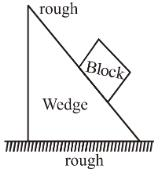Solution:

Friction force between wedge and block is internal i.e. will not change motion of centre of mass. Friction force on the wedge by ground is external and causes centre of mass to move towards right. Gravitational force (mg) on block brings it downward hence centre of mass comes down.

The correct answer is: rightward and downward

*Multiple options can be correct
QUESTION: 2

### Assuming potential energy U at ground level to be zero.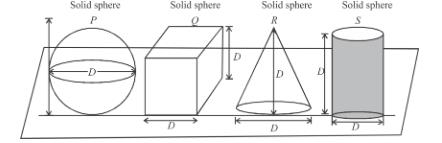All objects are made up of same material UP = Potential energy of solid sphere UQ = Potential energy of solid cube UR = Potential energy of solid cone US = Potential energy of solid cylinder

Solution: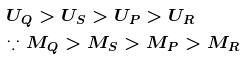centre of mass of cone is on smallest height.

The correct answers are: US > UPUQ >USUS > UR

*Multiple options can be correct
QUESTION: 3

### Two particles A and B start moving due to their mutual interaction only. If any time t,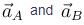are their respective acceleration,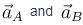are their respective velocities and upto that time WA and WB are the work done on A and B respectively by the mutual force, mA and mB are their masses respectively, then which of the following is always incorrect.

Solution:

Since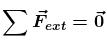∴  Momentum of system will remain conserved, equal to zero.

The correct answers are: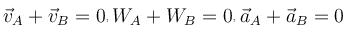*Multiple options can be correct
QUESTION: 4

The diagram shows the velocity-time graph for two masses  R and S that collided elastically. Which of the following statement is true?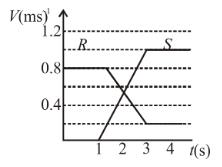Solution: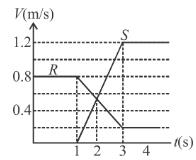(i) Since, both have positive final velocities, hence, both moved in the same direction after collision.

(ii) at t = 2 sec. both had equal velocities.

(iii) by conservation of linear momentum, we can say that mass of R was greater than mass of S.

The correct answers are: R and S moved in the same direction after the collision, The velocities of R and S were equal at the mid time of the collision, The mass of R was greater than mass of S

*Multiple options can be correct
QUESTION: 5

A particle strikes a horizontal smooth floor with a velocity u making an angle  with the floor and rebounds with velocity v making and angle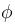with the floor. If the coefficient of restitution between the particle and the floor is e,  then :

Solution: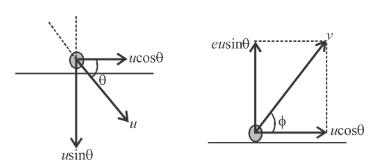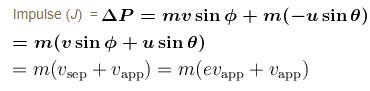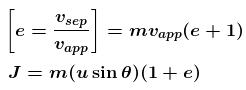In horizontal direction, momentum is conserved :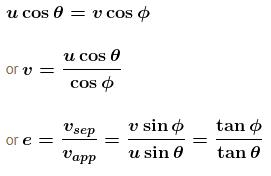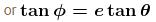in vertical direction,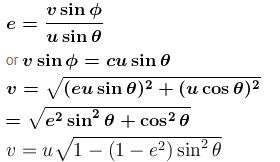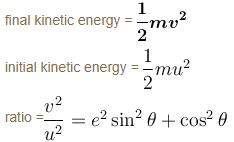The correct answers are: the impulse delivered by the floor to the body is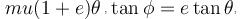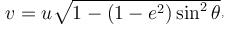the ratio of the final kinetic energy to the initial kinetic energy is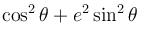*Multiple options can be correct
QUESTION: 6

A bag of mass M hangs by a long thread and a bullet (mass m) comes horizontally with velocity v and gets caught in the bag. Then for the combined system (bag + bullet) which of the following are correct

Solution:

Since, Fext = 0
Hence, momentum will remain conserved equal to mv.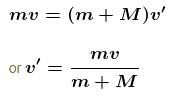and final kinetic energy is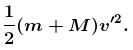The correct answers are: Momentum is mv, KE is m2v2(M + m)/2

*Multiple options can be correct
QUESTION: 7

A ball of mass m moving with a velocity v hits a massive wall of mass M (M >> m) moving towards the ball with a velocity u. An elastic impact lasts for a time  Δt. Which of the following are correct.

Solution:

In an elastic collision
vsep = vapp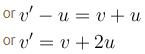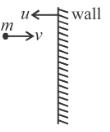change in momentum of ball is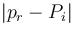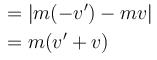= 2m(u + v)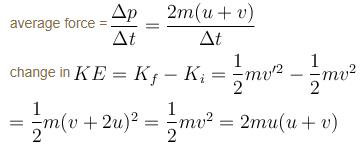The correct answers are: The average elastic force acting on the ball is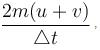The kinetic energy of the ball increase by 2mu(u+v)

*Multiple options can be correct
QUESTION: 8

Two blocks of equal mass m are connected by an unstretched spring and the system is kept at rest on a frictionless horizontal surface. A constant force F is applied on the first block pulling it away from the other as shown in figure.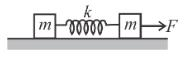Solution:

The acceleration of the centre of mass is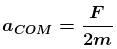The displacement of the centre of mass at time t will be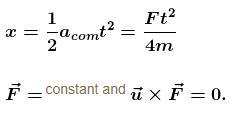Therefore initial velocity is either in direction of constant force or opposite to it. Hence the particle will move in straight line and speed may increase or decrease. When F and u are antiparallel then particle will come to rest for an instant and will return back.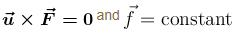Initial velocity is perpendicular to constant force, hence the path will be parabolic with speed of particle increasing.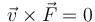means instantaneous velocity is always perpendicular to force. Hence the speed will remain constant. And also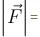constant. Since the particle moves in one plane, the resulting motion has to be circular.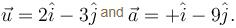Hence initial velocity is in same direction of constant acceleration, therefore, particle moves in straight line with increasing speed.

The correct answers are: The displacement of the centre of mass at time t is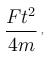The displacement of the right block, if the extension of the spring is x0 at time t, is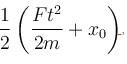The displacement of the left block, if the extension of the spring is x0 at time t, is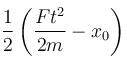*Multiple options can be correct
QUESTION: 9

For a two-body system in absence of external forces, the kinetic energy as measured from ground frame is K0 and from centre of mass frame is Kcm. Pick up the correct statement.

Solution:

It can be shown that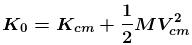where M is the total mass of the system and Vcm is velocity of centre of mass with respect to ground.

Due to internal changes Kcm can change but Vcm will remain same. Hence only Kcm portion of kinetic energy can be transformed to some other from of energy.
The correct answers are: The kinetic energy as measured from centre of mass frame is least, Only the portion of energy Kcm can be transformed from one form to another due to internal changes in the system., The system always retains at least K0Kcm amount of kinetic energy as measured from ground frame irrespective of any kind of internal change in the system.

*Multiple options can be correct
QUESTION: 10

A smooth sphere A of mass m collides elastically with an identical sphere B at rest. The velocity of A before collision is 8 m/s in a direction making 60° with the line joining the centre at the time of impact. Which of the following is/are possible :

Solution:

Sphere A moving with velocity v has a component v/2 along the line joining the centres of the spheres at the time of collision and another component   perpendicular to the previous direction. After collision the component along the line will interchange i.e. B will move with v/2 velocity i.e., 4 m/s along the line joining the centres and A moves with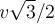velocity at perpendicular direction to B.

The correct answers are: the direction of motion of A and B after collision are at right angles, the speed of B after collision is 4 m/s

Track your progress, build streaks, highlight & save important lessons and more!

### Similar Content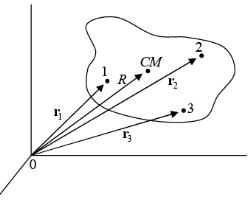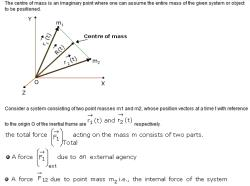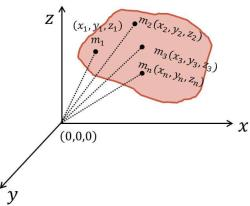### Related tests